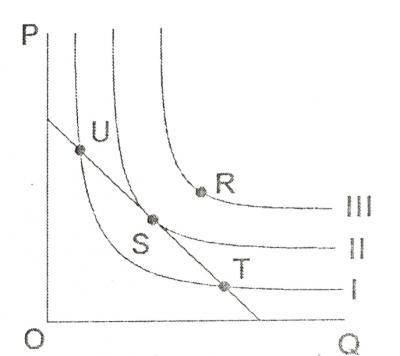# Economics 2018 Past Questions | JAMB

Study the following Economics past questions and answers for JAMBWAEC  NECO and Post-JAMB. Get prepared with official past questions and answers for upcoming examinations.

31.  An upward movement along the same supply curve result in_____________

• A. a decrease in price
• B. an increase
• C. an increase in quantity supplied
• D. a decrease In quantity supplied

32.  The coefficient of the price elasticity of supply is always_____________

• A. Constant
• B. Zero
• C. Positive
• D. Negative

33.  In the history of economics the concept of division of labour is usually associated With______________-

• A. Karl Marx
• C. David Ricardo
• D. J.M Keynes

34.  Given that Y = C + 1, where C = 50 + 0.75 and 1 = N45m, what is the equilibrium level of income?

• A. N20m
• B. N380m
• C. N200m
• D. N180m

35.From the diagram shown, the optional point of production is_____________?

• A. R
• B. T
• C. S
• D. U

36.  An ad valorem tax is imposed on_____?

• A. Exports
• B. Imports
• C. The Value of a Commodity
• D. Special Commodities

37.   The labour force of a country is determined by the___________

• A. geographical distribution of the population
• B. age structure of the population
• C. number of people available for work
• D. sex distribution of the population

38.  The transfer of public share holding in corporations to private enterprise is___________?

• A. Incorporation
• B. Concession
• C. Privatization
• D. Commercialization

39.  An economy in which both the public and private sectors contribute to economic growth is a__________?

• A. socialist economy
• B. feudal economy
• C. mixed economy
• D. capitalist economy

40.  A  capital market differs from the money market in that in the former ________?

• A. The percentage of interest charged is more
• B. Loan sought is long term
• C. Loan repayment is guaranteed
• D. Loan sought is short-term

## All Subjects

Subscribe
Notify ofInline Feedbacks